# Units - math word problems

1. Store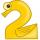Peter paid in store 3 euros more than half the amount that was on arrival to the store. When he leave shop he left 10 euros. How many euros he had upon arrival to the store?
2. Stairs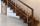Between adjacent levels are 15 stairs. If step will be 1.2 cm lower there would be 16 stairs. What is the stair height?
3. 9-gon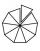Sum of interiol angles of 9-gon is:
4. Supplementary angles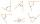One of the supplementary angles are three times larger than the other. What size is larger of supplementary angles?
5. Mr Novak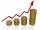Mr Novak earns an average 1249 euros. When increasing the salary they gave him a choice: ? What is better choice for Mr Novak?
6. Rhomboid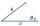The dimensions of the rhomboid sides are a= 5cm, b = 6 cm and the size of the angle at the vertex A is 60°. What is the length of side AC?
7. Grower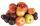Grower harvested 190 kg of apples. Pears harvested 10 times less. a) How many kg pears harvested? b) How many apples and pears harvested? c) How many kg harvested pears less than apples?
8. Aunt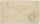Annie aunt is 35 years old, Annie is 14 years. For how many years will aunt have twice as many years as Annie?
9. BuingBrother got to buy 240 CZK and could buy for 1/8 what he wanted. Could he pay the rest of the purchase for 200 CZK?
10. Internal anglesOne internal angle of the triangle JAR is 25 degrees. The difference is the size of the two other is 15°. Identify the size of these angles.
11. Angles ratioIn a triangle ABC true relationship c is less than b and b is less than a. Internal angles of the triangle are in the ratio 5:4:9. The size of the internal angle beta is:
12. Little hand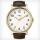What angle shifted little hand on the clock after one hour and 38 minutes?
13. Triangle anglesIn a triangle ABC the interior angle at the vertex C is twice as the internal angle at the point A. Outer angle at the point B measured 117 degrees. How big is the outer angle at the vertex A?
14. Invitations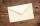For every 5 minutes writes Dana 10 invitations, while Anna 14 invitations. How long will write together 120 invitations?
15. The chordSide of the triangle inscribed in a circle is a chord passing through circle center. What size are the internal angles of a triangle, if one of them is 40°?
16. Garden pool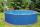Mr. Novak fill garden pool (cylinder; diameter of 200 cm) with 31.4 hl of water. What is the depth of the pool when the water level is 10 cm below the upper edge of the pool?
17. Clock hands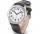The hands on the clock shows the time 12 hours and 2 minutes. Calculate the size of a sharp angle between clock hands three hours later.
18. Children in the classroom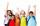How many children are in the classroom, where Ladislav is 10th lightest and 16th heaviest in class?
19. Clock Tower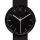What angle is betwenn hands on Clock Tower when show 17 hours and 35 minutes?
20. Water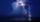On the lawn which has area 914 m2 rained 3 mm of water. How many liters of water rained?

Do you have an interesting mathematical word problem that you can't solve it? Submit math problem, and we can try to solve it.

We will send a solution to your e-mail address. Solved examples are also published here. Please enter the e-mail correctly and check whether you don't have a full mailbox.

Please do not submit problems from current active competitions such as Mathematical Olympiad, correspondence seminars etc...